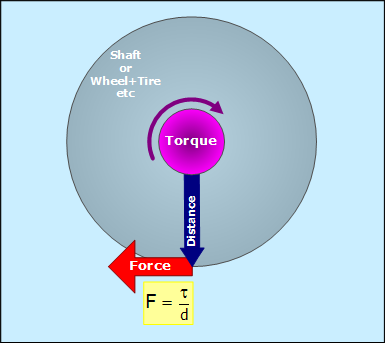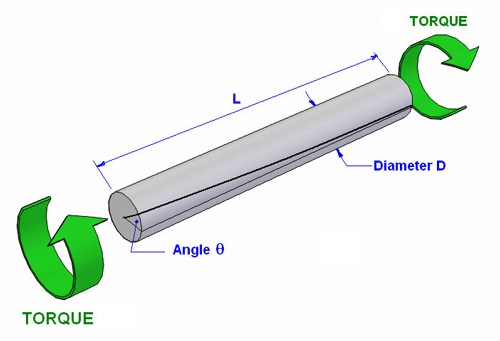# Difference Between Torque and Torsion

Torque is a parameter which can be measured directly, however twist is measured in terms of shear stress and the angle of twist.

Torque can be applied even if there is only one force acting on the object. However, twist needs at least two forces.

Torque does not depend upon the shape of the object, which is the case in twist. It also does not depend on the type of material and its failure characteristics.

### Instructions

• 1

Torque

Torque, moment or moment of force takes places when a force is applied to rotate an object, about a specific axis or reference point. Torques is always used to rotate the object and depends upon the magnitude of the force and the moment arm, i.e., the distance between the point of application of force and the point where the object is fixed. The moment arm is also called the lever arm. Another factor which affects the torque is the angles between the direction of the force and that of the lever arm.

The best example of a torque being applied on an object is when your rotate a fly wheel.

A force is applied to the fly wheel which rotates when the magnitude of the forces exceeds its weight. Denoted by the symbol τ, the Greek letter tau, torque is the phenomenon which produces a twist in the object. The SI unit for torque is the Newton meter (Nm).

Image courtesy: craig.backfire.ca• 2

Torsion

Torsion is the twisting of an object caused when torque is applied on it. Torsion normally takes place perpendicular to the longitudinal axis of the object and can result in permanent damage.

This property depends upon a number of factors including maximum shear stress at the outer surface of the object which suffers the twist, the torsion constant, distance between the axis and the farthest point on the object, length of the object, angle of twist and the shear modulus.

The SI unit for torsion is also Newton meter (Nm).

Image courtesy: ejsong.com•